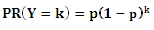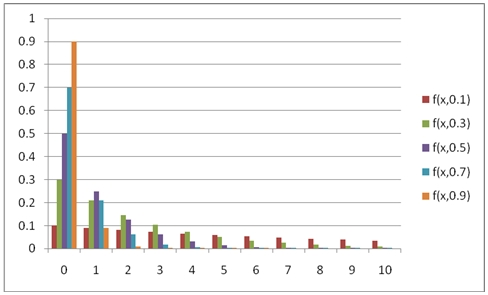# SQL Server geometric distribution function

GEOMETRIC

Updated: 31 July 2010

Use GEOMETRIC to calculate the probability mass function of the geometric distribution.

The formula for the probability mass function is:Syntax
SELECT [wctStatistics].[wct].[GEOMETRIC] (
<@X, float,>
,<@P, float,>)
Arguments
@X
is the number of failures. @X is an expression of type float or of a type that implicitly converts to float
@P
is the probability of success. @P is an expression of type float or of a type that implicitly converts to float
Return Types
float
Remarks
·         @X must be greater than or equal to zero (@X ≥ 0).
·         @P must be greater than zero and less than or equal to one (0 < @P ≤ 1).
·         @X is truncated to zero decimal places
·         For the lower cumulative distribution, use GEOMETRICP.

Examples
Calculate the probability mass function:
SELECT wct.GEOMETRIC(3,0.4)
This produces the following result
----------------------
0.0864

(1 row(s) affected)
You can use the SeriesInt function from the XLeratorDB/math library to generate a dataset which can be pasted into EXCEL to generate a graph of the probability mass function.
SELECT SeriesValue
,wct.GEOMETRIC(SeriesValue, 0.1) as [f(x,0.1)]
,wct.GEOMETRIC(SeriesValue, 0.3) as [f(x,0.3)]
,wct.GEOMETRIC(SeriesValue, 0.5) as [f(x,0.5)]
,wct.GEOMETRIC(SeriesValue, 0.7) as [f(x,0.7)]
,wct.GEOMETRIC(SeriesValue, 0.9) as [f(x,0.9)]
FROM wct.SeriesInt(0,10,NULL,NULL,NULL)
This is an EXCEL-generated graph of the results### SupportCopyright 2008-2021 Westclintech LLC         Privacy Policy        Terms of Service